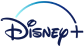## FANDOM

762 Pages

The gamelog is a file that logs activity over the entire game. Its main use currently is in ranking players on pubserver.

As of Freeciv 2.0, the gamelog format has changed. Below is the specification.

```<?xml version="1.0" encoding="UTF-8"?>
```

root element:

```<gamelog version="ver">
```

children:

```<map> the map </map>
```
```<player y="year" t="turn number">
<n> player number </n>
<u> user name </u>
<c> 1 if the player is connected to a user, 0 if not (observers are not) </c>
<ai> skill level name if AI, empty if human </ai>
<nat> nation name (plural) </nat>
</player>
```
```<team y="year" t="turn number">
<id> team id number </id>
<name> team name </name>
<n> player number (one for each player on team) </n>
</team>
```
```<treaty y="year" t="turn number">
<n1> the first player number in the treaty or the giver </n1>
<n2> the second player number in the treaty of the receiver </n2>
<city> if a city is transferred, the city name </city>
<type> the treaty clause name </type>
<m> message </m>
</treaty>

treaty clause can be one of: Embassy, Tech, Gold, Map, Seamap, City,
Ceasefire, Peace, Alliance, Team, Shared Vision
```
```<dipl y="year" t="turn number">
<n1> the first player number </n1>
<n2> the second player number </n2>
<type> the diplomatic state string </type>
<m> message </m>
</dipl>
```
```<tech y="year" t="turn number">
<n1> the player [number] receiving the tech </n1>
<n2> if present, the player [number] the tech received (or stolen) from </n2>
<name> the tech name </name>
<m> message </m>
</tech>
```
```<gov y="year" t="turn number">
<n> the player [number] forming the new government </n>
<name> the new government name </name>
<m> message </m>
</gov>
```
```<rev y="year" t="turn number">
<n> the player [number] revolting </n>
<m> message </m>
</rev>
```
```<cityf y="year" t="turn number">
<n> the player [number] of the founded city </n>
<name> the new city name </name>
<x> x coordinate </x>
<y> y coordinate </y>
<m> message </m>
</cityf>
```
```<cityl y="year" t="turn number">
<n1> the player [number] owning the city </n1>
<n2> the player [number] the city was lost to </n2>
<name> the lost city name </name>
<x> x coordinate </x>
<y> y coordinate </y>
<m> message </m>
</cityl>
```
```<cityd y="year" t="turn number">
<n> the player [number] of the disbanded city </n>
<name> the disbanded city name </name>
<x> x coordinate </x>
<y> y coordinate </y>
<m> message </m>
</cityd>
```
```<unitl y="year" t="turn number">
<n1> the player [number] losing the unit </n1>
<n2> if present, the player [number] killing the unit </n2>
<name> the unit type name </name>
<m> message </m>
</unitl>
```
```<gamel y="year" t="turn number">
<n> the player [number] losing the unit </n>
<name> the gameloss unit name </name>
<m> message </m>
</gamel>
```
```<embassy y="year" t="turn number">
<n1> the player [number] creating the embassy </n1>
<n2> the player [number] owning the city where the embassy was created </n2>
<name> the city name where the embassy was created </name>
<x> x coordinate </x>
<y> y coordinate </y>
<m> message </m>
</embassy>
```
```<build y="year" t="turn number">
<n> the player [number] building the unit or improvement </n>
<city> the name of the city </city>
<u> 1 if unit, 0 if not </u>
<w> 1 if improvement and wonder, 0 if not </w>
<name> name of unit or improvement </name>
<m> message </m>
</build>
```
```<geno y="year" t="turn number">
<n> the player [number] being killed </n>
<b> 1 if barbarian, 0 if not </b>
<m> message </m>
</geno>
```
```<rates y="year" t="turn number">
<n> the player [number] </n>
<tax> the new tax rate </tax>
<lux> the new luxury rate </lux>
<sci> the new science rate </sci>
</rates>
```
```<info y="year" t="turn number">
<n> the player [number] </n>
<cities> number of cities </cities>
<pop> total population </pop>
<food> total food </food>
<prod> total production </prod>
<settlers> number of settlers </settlers>
<units> number of units </units>
</info>
```
```<status y="year" t="turn number">
<plr>
<no> the player [number] </no>
<r> the rank </r>
<s> total population </s>
</plr>
...
</status>
```
```<judge y="year" t="turn number">
<type> the type of win </type>
<n> the player [number] winning </n>
...
<m> message </m>
</judge>

win type can be one of: None, Draw, Lone Win, Team Win, Allied Win
```
Community content is available under CC-BY-SA unless otherwise noted.